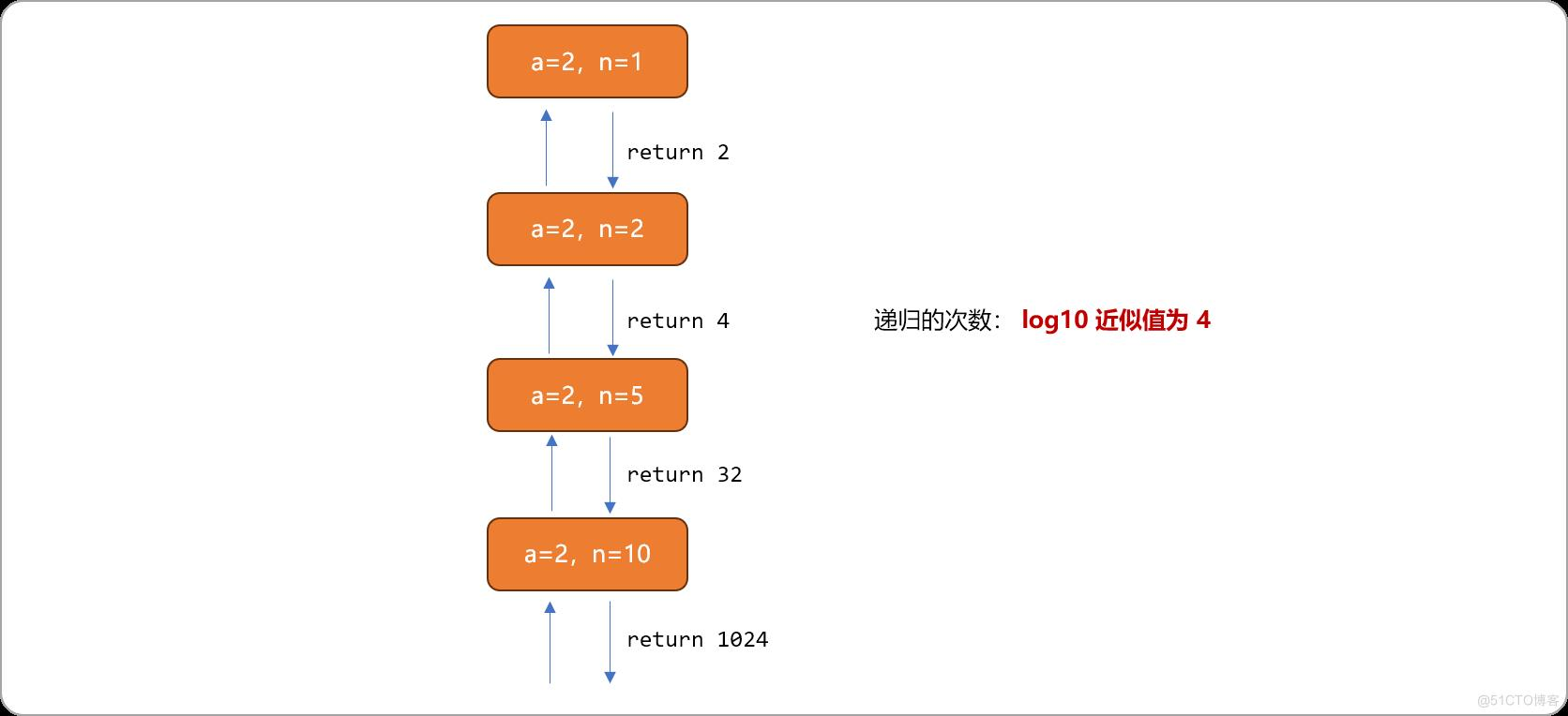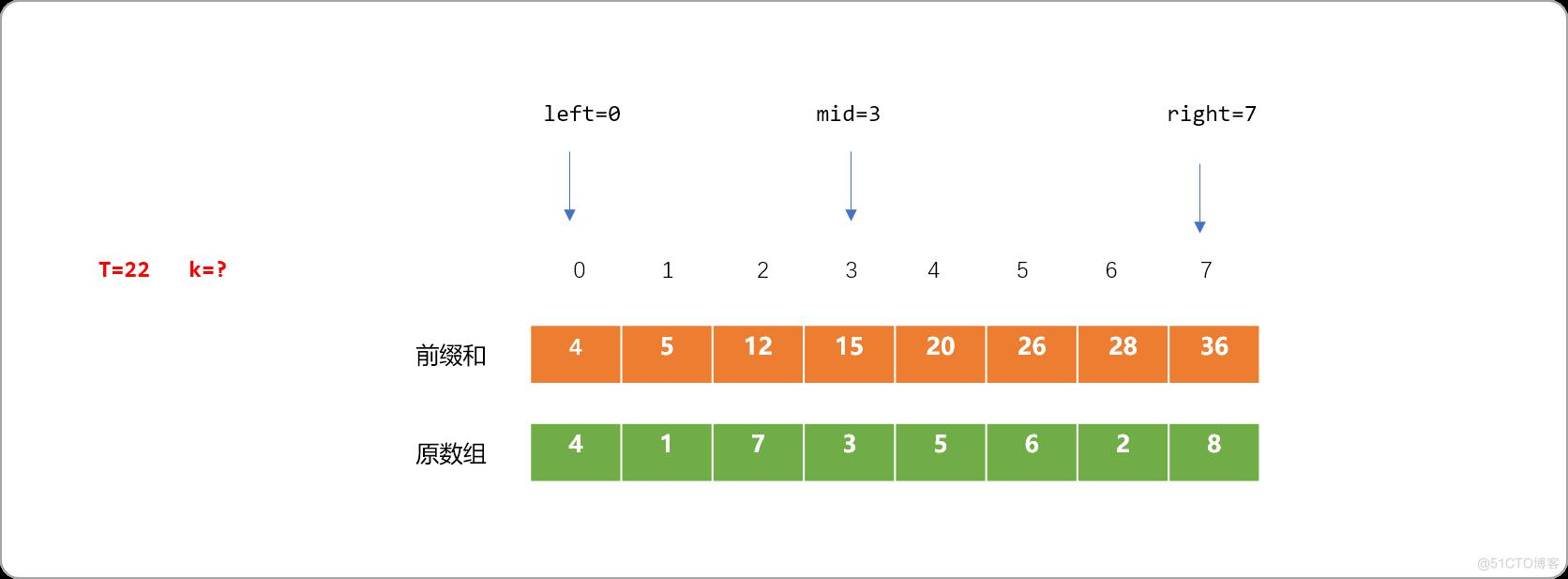﻿ C++算法进阶系列之倍增算法解决求幂运算 - 自由资讯

# C++算法进阶系列之倍增算法解决求幂运算

1. 引言 学习倍增算法，先了解什么是倍增以及倍增算法的优势。如果面前有一堆石子，要求计算出石子的总数量。 这是一个简单的数数问题，可以： 一颗石子一颗石子的数。 两颗石子

## 1. 引言

• 一颗石子一颗石子的数。
• 两颗石子两颗石子的数。
• 三颗石子三颗石子的数。
• 或者更多颗石子更多颗石子的数……

`17=16+1；13=8+4+1；……`

## 2. 快速幂

``````#include <iostream>
using namespace std;
int main(int argc, char** argv) {
int a,n;
cin>>a>>n;
//初始化累乘结果
int res=1;
for(int i=0;i<n;i++){
res*=a;
}
cout<<res<<endl;
return 0;
}
``````

### 2.1 转换一

• `n` 为偶数时， `a`<sup>n</sup>=`(a`<sup>n/2</sup>`)`<sup>2</sup>。
• `n` 为奇数时， `a`<sup>n</sup>=`(a`<sup>n/2</sup>`)`<sup>2</sup>*`a`<sup>n%2</sup>。

``````#include <iostream>
using namespace std;
long long int ksm(int  a,int n) {
long long int ans=1;
if(a==1)return 1;
if(n==1)return a;
ans=ksm(a ,n/2);
ans*=ans;
if(n%2==1) //如果是奇数
ans*=a;
return ans;
}
int main(int argc, char** argv) {
int a,n;
cin>>a>>n;
long long int ans=ksm(a,n);
cout<<ans;
return 0;
}
``````### 2.2 转换二

a<sup>255</sup>=`*a`<sup>128</sup>`*a`<sup>64</sup>`*a`<sup>32</sup> `*a`<sup>16</sup>`*a`<sup>8</sup>`*a`<sup>4</sup>`*a`<sup>2</sup>`*a`<sup>1</sup> ；

• a<sup>1</sup>=a。
• a<sup>2</sup>=a<sup>1</sup>*a<sup>1</sup>。
• a<sup>4</sup>=a<sup>2</sup>*a<sup>2</sup>。
• a<sup>8</sup>=a<sup>4</sup>*a<sup>4</sup>。
• ……
• a<sup>128</sup>=a<sup>64</sup>`*a`<sup>64</sup>。

• 求解出 a<sup>1</sup>的值: `a`<sup>1</sup>=`a`。因为 `1010`的最后一位为 `0`，a<sup>1</sup> 的值不累乘到最终结果中。因为要使用 a<sup>1</sup> 推导出 a<sup>2</sup> 的值，前面 a<sup>1</sup> 的值需要存储，在整个求值过程，可以设置 2 个变量，一个用来存储一路推导出来的值，即`a`<sup>i</sup>`（i=1，2，4，8）`，一个用来存储最终结果值。
``````//存储迭代值
int base =a;
//存储最终结果值
int res=1;
if( 1010 的最后一位是 0 )
base 的值不能被累乘到 res 中。
``````
• 第二次迭代，`base=base*base`，迭代出 a<sup>2</sup> 的值，因为`1010`的倒数第 `2` 位的值为 `1`。把此次 base 中的值累乘到 `res`
``````//第二次迭代
base *=base;
if( 1010的倒数第二位是 1 )
把 base 的值累乘到 res 中。
res*=base
``````
• 第三次迭代，`base=base*base`，迭代出 a<sup>4</sup> 的值，因为 `1010`的倒数第 3 位的值为 0，此次迭代的值不能累乘到 `res` 中。
``````//第三次迭代
base *=base;
if( 1010的倒数第三位是 0 )
base 中的值不能累乘到 res 中。
``````
• 第四迭代，`base=base*base`，迭代出 a<sup>8</sup> 的值，因为`1010`的倒数第 `1` 位的值为 `1`。把此次 base 中的值累乘到 `res`
``````//第四次迭代
base *=base;
if( 1010的倒数第四位是 1 )
base 值需要累乘到 res 中。
res*=base
``````

• 指数转化成二进制后，二进制有多少位，需要迭代多少次。
• 每次迭代都会得到一个中间值（存储在 `base`中），至于此值需不需要累乘到最终结果中，则需要根据二进制中的 `0``1`决定。如果是 `0`则不需要，`1`则需要。

``````#include <iostream>
#include <stack>
using namespace std;

/*
* 将数字转换成二进制字符串
*/
string binary(int num) {
string s="";
while( num>0 ) {
s+=num % 2+'0';
num=num/2;
}
return s;
}
/*
*快速幂
*/
int ksm(int a,int n) {
string s= binary(n);
//字符串二进制的长度
int len=s.length();
//初始化推导数
int base=a;
//结果
int res=1;
if(s=='1')
//如果最后一位是 1 ，则初始化为推导数
res*=base;
for(int i=1; i<len; i++) {
base*=base;
if(s[i]=='1')res*=base;
}
return res;
}

int main(int argc, char** argv) {
int a,n;
cin>>a>>n;
int res= ksm(a,n);
cout<<res;
return 0;
}
``````

• 每次迭代时，把`n=1010``1`相与`(n&1)`，可判断最后一位是是 `1`还是 `0`。位运算法则，`1``1`相与为`1``0``1`相与为`0`
• 当前迭代结束时，把`n=1010`向右移一位`(n>>1)`，则 `n=101`。为下一次迭代做准备。
• 重复上述两步，直到 `n=0`
``````//使用位运算符实现快速幂
int ksm(int a,int n) {
int base=a;
int res=1;
while(n>0) {
if(n & 1==1 ) {
//如果 n　最后一位是 1
res*=base;
}
base*=base;
//右移
n=n>>1;
}
return res;
}
``````

## 3. 前缀和

• 对原数列预处理，求出前缀和。• 如输入 T=22，求 `k`。可使用二查找算法。``````#include <iostream>
#include <stack>
using namespace std;
int main() {
//原数组
int nums= {4,1,7,3,5,6,2,8};
//前缀和数组
int sum= {0};
for(int i=0; i<8; i++) {
if(i==0)sum[i]=nums[i];
else sum[i]=sum[i-1]+nums[i];
}
int t,k;
cin>>t;
int left=0,right=7;
int midPos,midVal;
//二分查找
while(left<=right) {
midPos=(right+left)>>1;
midVal=sum[midPos];
if( midVal<=t )left=midPos+1;
else right=midPos-1;
}
k=right;
cout<<"最大的位置:"<<k<<endl;;
return 0;
}
``````

``````#include<iostream>
using namespace std;
int main() {
//原数组
int nums= {4,1,7,3,5,6,2,8};
//前缀和数组
int sum= {0};
for(int i=0; i<8; i++) {
if(i==0)sum[i]=nums[i];
else sum[i]=sum[i-1]+nums[i];
}
int k=0,i=1,s=0,t;
cin>>t;
while(i!=0) {
if(s+sum[k+i]-sum[k]<=t && sum[k+i]-sum[k]>0) {
s+=sum[k+i]-sum[k];
k+=i;
i<<=1;
} else i>>=1;
}
printf("%lld\n",k);
return 0;
}
``````

﻿